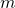# Approximation of zeros of m-accretive mappings, with applications to Hammerstein integral equations

### Abstractcarpathian_2020_36_1_59_69_abstract

An algorithm for approximating zeros of-accretive operators is constructed in a uniformly smooth real Banach space.
The sequence generated by the algorithm is proved to converge strongly to a zero of an-accretive operator.
In the case of a real Hilbert space, our theorem complements the celebrated proximal point algorithm of Martinet and Rockafellar for approximating zeros of maximal monotone operators. Furthermore, the convergence theorem proved is applied to approximate a solution of a Hammerstein integral equation. Finally, numerical experiments are presented to illustrate the convergence of our algorithm.